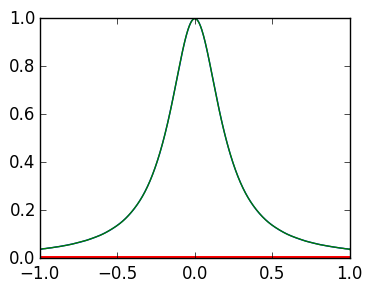# Abstract types¶

An abstract type represents groups of other types, but can never be instantiated on its own. For example, every integer and floating point number is a real number. Therefore, there is an abstract type Real, which encapsulates many other types, including Float64, Float32, Int64 and Int32.

We can test if type T is part of an abstract type V using the sytax T <: V:

In :
Float64 <: Real

Out:
true
In :
Float32 <: Real

Out:
true
In :
Int64 <: Real

Out:
true
In :
Int32 <: Real

Out:
true

As a counter example, complex numbers are not real, and therefore Complex types are not <: Real:

In :
C=typeof(1+im)   # returns Complex{Int64} or Complex{Int32}, depending on the machine

C <: Real    # Complex numbers are not real!

Out:
false

## Super types¶

Every type has one and only one super type, which is always an abstract type. The function super applied to a type returns its super type:

In :
super(Int32)  # returns Signed, which represents all signed integers.

Out:
Signed
In :
super(Int64)  # all returns Signed

Out:
Signed
In :
super(Float32)

Out:
AbstractFloat
In :
super(Float64)

Out:
AbstractFloat

An Abstract type also has a super type:

In :
super(Real)

Out:
Number
In :
super(Number)

Out:
Any
In :
super(Signed)

Out:
Integer
In :
super(Integer)

Out:
Real
In :
super(UInt32)

Out:
Unsigned
In :
super(AbstractFloat)

Out:
Real
In :
super(Number)

Out:
Any

Any is the largest type that contains every other type, and its super type is itself:

In :
super(Any)

Out:
Any

# Abstract types and defining functions¶

We can use abstract types to define functions, restricting the definition to only apply for types which are subtypes of the abstract type. For example, the following defines differently depending on whether x is an Integer or an AbstractFloat.

In :
function myfactorial(x::Integer)
factorial(x)
end
function myfactorial(x::AbstractFloat)
gamma(x+1)
end

Out:
myfactorial (generic function with 2 methods)
In :
myfactorial(5)   # 5 is an Int64, which is <: Integer, hence the first definition is called

Out:
120
In :
myfactorial(UInt32(5))   # UInt32(5) is a UInt64, which is <: Integer, hence the first definition is called

Out:
120
In :
myfactorial(5.5)  # 5.5 is a Float64, which is <: AbstractFloat, hence the second definition is called

Out:
287.88527781504433
In :
myfactorial(5.5f0)  # 5.5f0 is a Float32, which is <: AbstractFloat, hence the second definition is called

Out:
287.88528f0

# Abstract types and vectors¶

There are many different types that represent vectors. This property is captured using AbstractVector. For example, the command rand(5) returns a Vector{Float64}.

In :
v=rand(5)
typeof(v)==Vector{Float64}

Out:
true

On the other hand, the syntax a:b returns something that acts like a vector, but is not a vector:

In :
r=2:6
r

Out:
3
In :
typeof(r)==Vector{Int64}

Out:
false

Both v and r are AbstractVectors:

In :
typeof(v) <: AbstractVector{Float64}

Out:
true
In :
typeof(r) <: AbstractVector{Int64}

Out:
true

Any AbstractVector can be converted to a Vector using the function collect:

In :
r=2:7
collect(r)

Out:
6-element Array{Int64,1}:
2
3
4
5
6
7
In :
typeof(collect(r)) == Vector{Int64}

Out:
true

Big difference: the entries of Vectors can be changed, but AbstractVectors cannot, in general.

In :
v=rand(5)
v=3
v

Out:
5-element Array{Float64,1}:
0.586114
3.0
0.0513032
0.332904
0.440058 
In :
r=2:6
r=3   # Not allowed

LoadError: indexed assignment not defined for UnitRange{Int64}

in setindex! at abstractarray.jl:592

Use collect to make an AbstractVector changeable:

In :
r=collect(2:6)
r=3   # Allowed
r

Out:
5-element Array{Int64,1}:
2
3
4
5
6

## Interpolation¶

We saw last lecture that interpolating at evenly spaced points, runs into issues:

In :
using PyPlot

## this function evaluates a Taylor polynomial at a point x, or vector of points x, where c is a vector of coefficients.
function p(c,x)
n=length(c)
ret=0.0
for k=1:n
ret=ret+c[k]*x.^(k-1)   # use .^ instead of ^ to allow x to be a vector
end
ret
end

g=linspace(-1.,1.,100)    # plotting grid: 100 points between -1 and 1

plot(g,1./(25g.^2+1))     # plot the true function in blue

n=20                      # interpolate at 20 points
x=linspace(-1.,1.,n)
V=Float64[x[k]^(j-1) for k=1:n,j=1:n]    # construct the Vandermonde matrix

f=1./(25x.^2+1)         # evaluate the true function at the interpolation points
c=V\f                   # calculate the coefficients of the interpolating polynomial
plot(g,p(c,g))          # plot the interpolating polynomial be evaluating at the plotting grid ;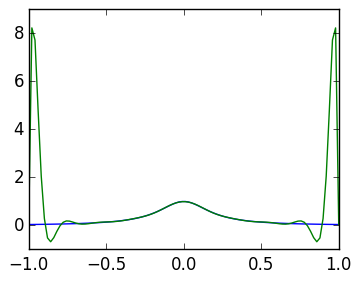Out:
1-element Array{Any,1}:
PyObject <matplotlib.lines.Line2D object at 0x30fda3410>

The "best" way would be to choose different interpolation points. A particularly good choice is as follows:

In :
n=50
θ=linspace(0,π,n)
x=cos(θ)

plot(x,zeros(n);marker=".",linestyle="");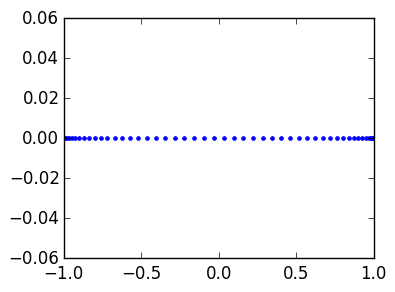In :
n=100
θ=linspace(0,π,n)
x=cos(θ)   # this is my new x, called "Chebyshev points"

V=Float64[x[k]^(j-1) for k=1:n,j=1:n]
f=1./(25x.^2+1)
c=V\f   # calculate the new coefficients at new points

plot(g,1./(25g.^2+1))
plot(g,p(c,g));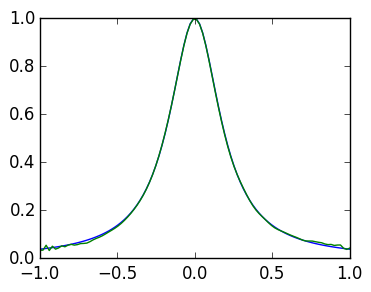Note there are still issues: we fixed the grid, but we also have to choose a better basis. We will come back to this later.

## Least squares¶

But what if we only know the function at evenly spaced points? We can still reliably approximate the function by using more points than coefficients.

In :
g=linspace(-1,1,1000)

plot(g,1./(25g.^2+1))  # plot the true function

m=20   # number of coefficients
n=50  # number of points

x=linspace(-1.,1.,n)   # use n evenly spaced points
V=Float64[x[k]^(j-1) for k=1:n,j=1:m]    # make an n x m Vandermonde matrix

f=1./(25x.^2+1)     # f evaluated at the m points
c=V\f    # find c so that V*c is approximately f

plot(g,p(c,g))
plot(x,zeros(n);marker=".",linestyle="")  # plot the grid;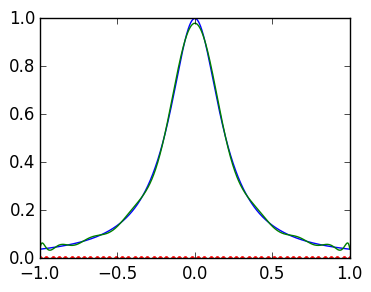\ only solves approximately: V*c is not exactly f:

In :
norm(V*c-f)

Out:
0.05488784046826881

Increasing the coefficients and number of points appropraitely improves the accuracy:

In :
g=linspace(-1,1,1000)

plot(g,1./(25g.^2+1))  # plot the true function

m=80   # number of coefficients
n=m^2  # number of points

x=linspace(-1.,1.,n)   # use n evenly spaced points
V=Float64[x[k]^(j-1) for k=1:n,j=1:m]    # make an n x m Vandermonde matrix

f=1./(25x.^2+1)     # f evaluated at the m points
c=V\f    # find c so that V*c is approximately f

plot(g,p(c,g))
plot(x,zeros(n);marker=".",linestyle="");